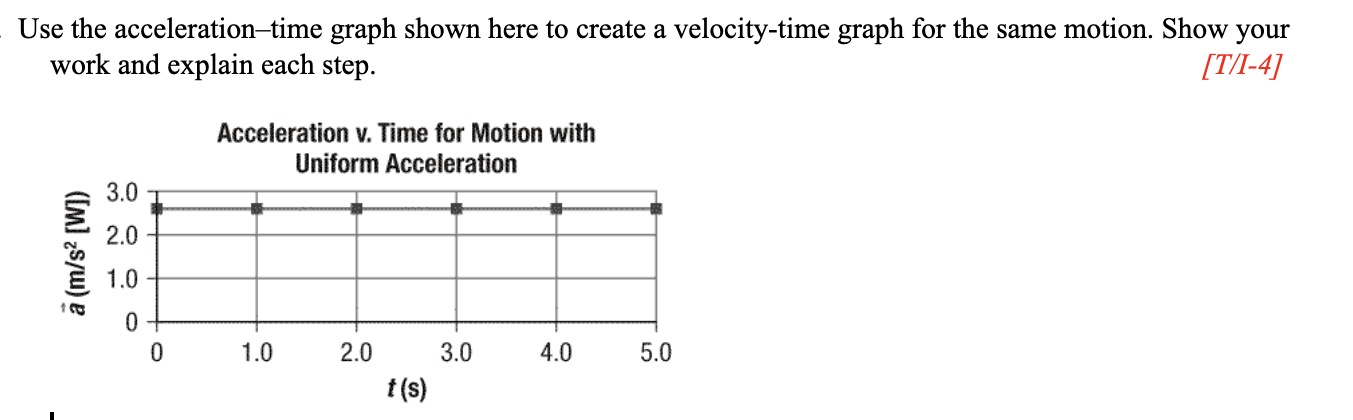# Question Solved1 AnswerUse the acceleration–time graph shown here to create a velocity-time graph for the same motion. Show your work and explain each step. Use the acceleration-time graph shown here to create a velocity-time graph for the same motion. Show your work and explain each step. [T/I-4] Acceleration v. Time for Motion with Uniform Acceleration 3.0 2.0 à (m/s? [W]) 1.0 0 0 1.0 2.0 3.0 4.0 5.0 t(s)Use the acceleration–time graph shown here to create a velocity-time graph for the same motion. Show your work and explain each step.Transcribed Image Text: Use the acceleration-time graph shown here to create a velocity-time graph for the same motion. Show your work and explain each step. [T/I-4] Acceleration v. Time for Motion with Uniform Acceleration 3.0 2.0 à (m/s? [W]) 1.0 0 0 1.0 2.0 3.0 4.0 5.0 t(s)
More
Transcribed Image Text: Use the acceleration-time graph shown here to create a velocity-time graph for the same motion. Show your work and explain each step. [T/I-4] Acceleration v. Time for Motion with Uniform Acceleration 3.0 2.0 à (m/s? [W]) 1.0 0 0 1.0 2.0 3.0 4.0 5.0 t(s)